Mobile QR Code1. (Department of Electronics, Guizhou University, Guiyang 550025, China)
2. (Key Laboratory of Micro-Nano Technology of Guizhou Province, Guiyang 550025, China)
3. (Engineering Research Center of Reliability of Semiconductor Power Devices of the Ministry of Education, Guiyang 550025, China)

Through-silicon-via, thermal model, thermal-mechanical reliability, finite element analysis

## I. INTRODUCTION

Three-dimensional (3D) integration gains more and more attention for its advances in high density integration, low power consuming, low signal delay and thus improved frequency performance (1,2). However, thermal issues are becoming critical for the reliability of the whole electronic system resulting from the 3D stacked structure which increases the power density (3-5). Through Silicon Vias (TSVs) inserting is proved to be an efficient method to mitigate the thermal problems for the high thermal conductivity of the Cu pillar inside a TSV structure experimentally (6). In a 3D stacked system, heat flows across different materials with disparate thermal properties which return makes the thermal path and the calculation of thermal resistance much more complicated. For simplicity, many publications only take vertical resistance (1D resistance in z-direction) into considerations to give a first insight of the thermal performance of 3D integrated systems and easily estimate the thermal management requirements (7-9). Though lateral thermal resistance is also considered (10-12), this kind of result is based on specific conditions that the heat source is perpendicular to the TSVs which is not usually the case in reality. Recently, the thermal resistance of a 3D laminated packaging structure is given based on simulation results but lacking of detailed physical derivations which is not suitable for the guidance of thermal management in 3D integrated systems (13). Due to the obvious coeffcients of thermal expansion (CTE) mismatch between the Si and filled-in-via materials, the resulting thermal stress could degrade the chip performance under thermal cycling, because the carrier mobility has been changed (14-16) and certain keep-out zone (KOZ) needs to be considered for thermal TSVs (TTSVs) application.

Fig. 1. Top view and cross section view of the power chip with TTSVs. The width and length of a power cell and bulk Si is a and b, respectively. And the distance between the nearest neighbor two TSVs is noted as P. The height of bulk Si and bonding layer in the 1st and 2nd layer is H1 and tb1, H2 and tb2, respectively. Parameters r, d is the radius of TSV, SiO$_{2}$ liner thickness, respectively.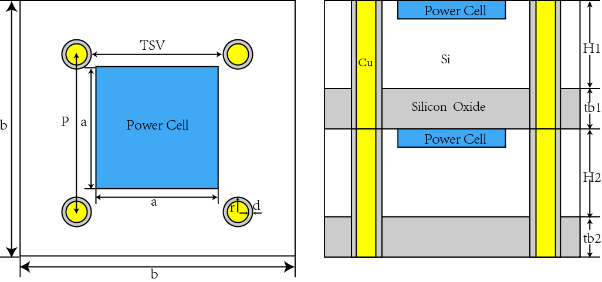In this paper, an analytical heat transfer model with transverse and longitudinal thermal resistance for TTSVs is proposed. In TTSVs, thermal stress impact on carrier mobility in active areas based on physical heat transfer analysis and simulation results is considered. Compared with the published 1D model, thermal properties, obtained by varying geometrical parameter of the TSV and related materials, of the proposed analytical heat transfer model are much closer to the corresponding Finite Element Analysis (FEA) simulated results. Therefore, the proposed analytical heat transfer model is valuable for guiding thermal design of 3D integrated systems. And KOZ consideration improves the thermal-mechanical reliability of the whole thermal mitigation system. At last, a case study of a two-layer stacked power system containing TTSVs is given to verify the accuracy and efficiency of the analytical model.

## II. ANALYTICAL HEAT TRANSFER MODEL

The proposed structure of TTSVs-based two-layer stacked power system is shown in Fig. 1. Power cells are abstractions of real power devices. During the operation, devices generate heat behaving as heat sources and temperature also increases for Joule heating. So, a power cell can be set to a unit cell which is surrounded by four Cu-TSVs which act as auxiliary heat dissipation paths.

Firstly, the KOZ when TSVs are used to conduct heat is derived. The mobility change of carriers caused by thermal stresses of TTSV can be expressed by

Fig. 2. (a) and (b) are top and cross section view of FEA model, respectively.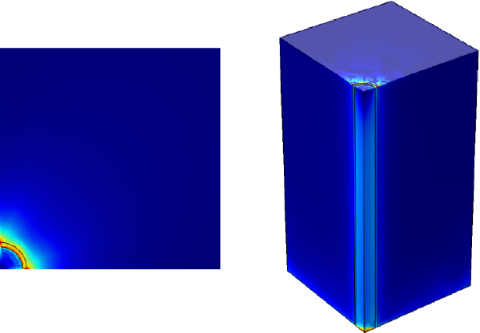Fig. 3. Critical boundary for various filling-via radius.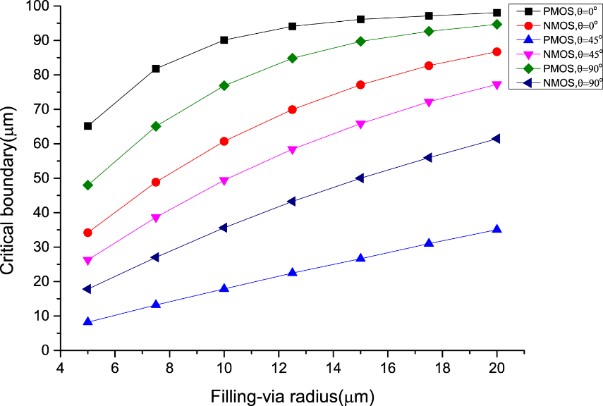##### (1)
$$\frac{\Delta \mu}{\mu}(r, \theta)=\pi \times \sigma_{n} \times \beta(\theta)$$

where ${\pi}$ is the piezoresistive coefficient, ${\sigma}$$_{rr} is the TSV-induced radial stress and {\beta}({\theta}) is the orientation factor. The radial stress distribution can be obtained by FEA simulation and the simulation model is constructed as shown in Fig. 2. For reducing the computation time, only one quarter of the TSV structure is built due to the symmetry in axial directions. Based on the 5% carrier mobility change criterion and Eq. (1), the critical boundary of KOZ under 380K thermal load for TSVs with filling-via radius varies from 5 {\mathrm{\mu}}m to 20 {\mathrm{\mu}}m, and 1~{\mathrm{\mu}}m dielectric layer thickness is plot in Fig. 3, where {\theta} is the angle between transistor’s channel and radial stress. As we can see, for PMOSFET, when {\theta} is 0^{\mathrm{o}}(TSV-induced stress is parallel to PMOS channel), the critical boundary of KOZ has maximum value. As the radius of filling-via increases, the corresponding KOZ boundary goes up. The distance between the nearest two neighbor TSVs is big enough in the simulation model. Therefore, the effect of interaction between TSVs on the KOZ can be ignored. Fig. 4. Cross section view of detailed thermal flux in diagonal direction of the heat mitigation structure.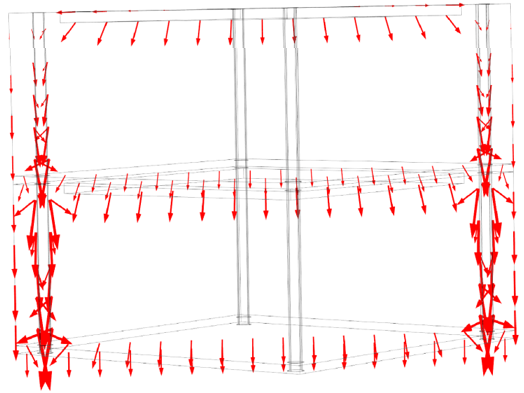Fig. 5. Thermal resistance network model for heat mitigation structure corresponding to Fig. 4.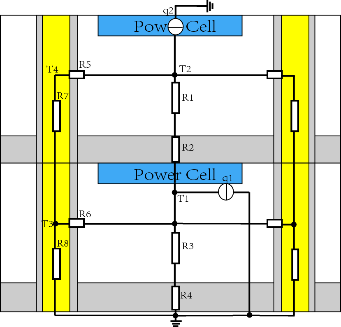There are mainly two paths for heat flowing from the power cell to the ambient. The first one is from the power cell to the bulk silicon and then to the bonding layer. Another one is from the power cell to the TSV structure. Most of heat dissipate from the TSV owing to the high thermal conductivity of the Cu pillar and there is still some heat dissipating from Cu pillar to the bulk silicon. The comparability of heat transfer and current flow can be applied for thermal analysis in a simple or more complex system. From the analysis of thermal path in the previous case, a detailed thermal flow diagram which also has been verified by FEA simulation shown in Fig. 4 and a thermal resistance network shown in Fig. 5 can be derived, respectively. As shown in the thermal resistance network, two heat sources are replaced by current sources q1 and q2. Similar to Kirchhoff's Circuit Law and Kirchhoff's Voltage Law in electronic circuits, we can derive ##### (2)$$ q_{1}=\frac{T_{1}}{R_{3}+R_{4}}+\frac{T_{1}-T_{3}}{R_{6}}-\frac{T_{2}-T_{1}}{R_{1}+R_{2}} $$##### (3)$$ q_{2}=\frac{T_{2}- T_{1}}{R_{1}+R_{2}}+\frac{T_{2}- T_{4}}{R_{5}} $$##### (4)$$\frac{T_{2}- T_{4}}{R_{5}}=\frac{T_{4}- T_{3}}{R_{7}}$$##### (5)$$ \frac{T_{1}- T_{3}}{R_{6}}+\frac{T_{4}- T_{3}}{R_{7}}=\frac{T_{3}}{R_{8}} $$Rearrange equations from (2)-(5), yields ##### (6)$$ \left(\frac{1}{R_{3}+R_{4}}- \frac{1}{R_{1}+R_{2}}+\frac{1}{R_{6}}\right)T_{1}- \frac{1}{R_{1}+R_{2}}T_{2}- \frac{1}{R_{6}}T_{3}=q_{1} $$##### (7) -$$ \frac{1}{R_{1}+R_{2}}T_{1}+\left(\frac{1}{R_{1}+R_{2}}+\frac{1}{R_{5}}\right)T_{2}- \frac{1}{R_{5}}T_{4} =q_{2} \\ $$##### (8)$$ \frac{1}{R_{5}}T_{2}+\frac{1}{R_{7}}T_{3}- \left(\frac{1}{R_{5}}+\frac{1}{R_{7}}\right)T_{4} =0 \\ $$##### (9)$$ \frac{1}{R_{6}}- \left(\frac{1}{R_{6}}+\frac{1}{R_{7}}+\frac{1}{R_{8}}\right)T_{3}+\frac{1}{R_{7}}T_{4} =0 $$Thermal resistance is proportional to the thermal path length and inverse proportional to the product of thermal conductivity of the material and area of the thermal path, which is ##### (10)$$ R=\frac{L}{\kappa \cdot S} $$Then thermal resistance for each element is giving as follows: ##### (11)$$ R_{1} =\frac{\left(b- a\right)/2}{\kappa _{Si}\left(\frac{a+b}{2}\right)^{2}}+\frac{H_{1}- \left(b- a\right)/2}{\kappa _{Si}\left(b^{2}- 4\pi r^{2}\right)} \\ $$##### (12)$$ R_{2} =\frac{tb_{1}}{\kappa _{Si{O_{2}}}\left(b^{2}- 4\pi r^{2}\right)} \\ $$##### (13)$$ R_{3} =\frac{\left(b- a\right)/2}{\kappa _{Si}\left(\frac{a+b}{2}\right)^{2}}+\frac{H_{2}- \left(b- a\right)/2}{\kappa _{Si}\left(b^{2}- 4\pi r^{2}\right)} \\ $$##### (14)$$ R_{4} =\frac{tb_{2}}{\kappa _{Si{O_{2}}}\left(b^{2}- 4\pi r^{2}\right)} \\ $$##### (15)$$ R_{5}=\frac{1}{4}\cdot 2\int _{r- \frac{d}{\cos \alpha }}^{r}\frac{dl}{\kappa _{Si{O_{2}}}\pi l\left(H_{1}+tb_{1}\right)}+\frac{\pi }{\kappa _{Cu}2\left(H_{1}+tb_{1}\right)}\\ =\frac{- \ln (1- \frac{d}{r\cos \alpha })}{2\pi \kappa _{Si{O_{2}}}\left(H_{1}+tb_{1}\right)}+\frac{\pi }{\kappa _{Cu}2\left(H_{1}+tb_{1}\right)} $$Fig. 6. Detailed schematic diagram of heat entering from bulk Si to TSV liner SiO_{2}.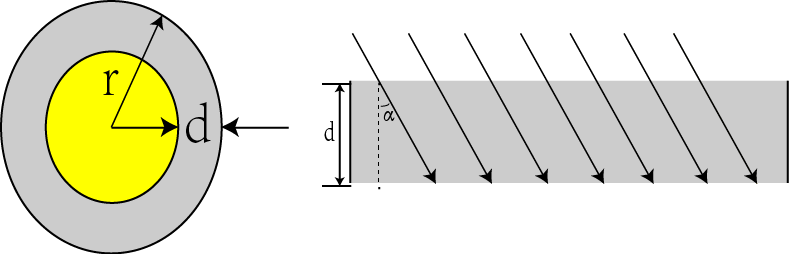##### (16)$$ R_{6}=\frac{1}{4}\cdot 2\int _{r- \frac{d}{\cos \alpha }}^{r}\frac{dl}{\kappa _{Si{O_{2}}}\pi l\left(H_{2}+tb_{2}\right)}+\frac{\pi }{\kappa _{Cu}2\left(H_{2}+tb_{2}\right)}\\ =\frac{- \ln (1- \frac{d}{r\cos \alpha })}{2\pi \kappa _{Si{O_{2}}}\left(H_{2}+tb_{2}\right)}+\frac{\pi }{\kappa _{Cu}2\left(H_{2}+tb_{2}\right)} $$##### (17)$$ R_{7} =\frac{1}{4}\cdot \frac{H_{1}+tb_{1}}{\kappa _{Cu}\pi (r- d)^{2}} \\ $$##### (18)$$ R_{8} =\frac{1}{4}\cdot \frac{H_{2}+tb_{2}}{\kappa _{Cu}\pi (r- d)^{2}} $$First terms on the right side of Eqs. (11) and (13) result from small heat source enters in bigger conduction area and leads to thermal spreading resistance. To simplify the complicated analytical expressions for the spreading resistance, usually the thermal spreading angle is chosen 45o. In Eqs. (15)-(18), the coefficients 1/4 results from the fact that one power cell is surrounded by four TTSVs and these TTSVs are in parallel in equivalent thermal network shown in Fig. 5. The lower and upper limit of integral in Eqs. (15) and (16) is depicted in Fig. 6. First and second term of Eqs. (15) and (16) represents transverse resistance of TSV liner and TSV filling-via, respectively. Heat flows from Si to TSV liner with specific angle α which actually enlarge the thermal path of the TSV liner transverse resistance. Substitute Eqs. (11)-(18) into Eqs. (6)-(9), temperature rise for each layer can be derived. Similar to Eqs. (2)-(18), the following relationships for 1D thermal resistance network shown in Fig. 7 can be derived, which are ##### (19)$$ \frac{- 1}{R_{11}+R_{22}}\cdot T_{1}+\frac{1}{R_{11}+R_{22}}\cdot T_{2}=q_{2} \\ $$##### (20)$$ \frac{1}{R_{33}+R_{44}}\cdot T_{1}=q_{1}+q_{2} $$where R_{11} and R_{33} are effective thermal resistance for the 1st and 2nd Si layer, and R_{22} and R_{44} are effective thermal resistance for the 1st and 2nd bonding layer. Their detailed expressions can be derived using effective thermal conductivity for each layer containing TSV structures. ## III. SIMULATING AND DISCUSSIONS Material for the bonding layer and TSV liner is silicon oxide and the filling-via material is Cu. Thermal conductivity for silicon, silicon oxide and copper, namely {\sigma}$$_{\mathrm{Si}}$, ${\sigma}$$_{\mathrm{SiO2}}, {\sigma}$$_{\mathrm{Cu}}$, is 130 W/(m${\cdot}$K), 1.38 W/(m${\cdot}$K), 400 W/(m${\cdot}$K),respectively. Angel ${\alpha}$ is also assumed to be 45$^{\mathrm{o}}$.We assume the power cell is composed of PMOS device and the angle ${\theta}$ is 45$^{\mathrm{o}}$. From Fig. 3 we can find that the minimum KOZ boundary for filling-via radius range from 5 ${\mathrm{\mu}}$m to 20 ${\mathrm{\mu}}$m is 35.08 ${\mathrm{\mu}}$m .The heat source power for each layer on the surface is 1W. The bottom surface adjacent to the heat sink is set 27 $^{\mathrm{o}}$C which assumes the heat exchange coefficient is approaching infinity for the heat sink. The rest of the surfaces are adiabatic. Fig. 8 to 11 shows the temperature difference with respect to the filling-via radius, thickness of bulk Si, TSV liner thickness and thickness of bonding layer, respectively. Meanwhile the proposed model is compared with the FEA simulation and the 1D model result to verify its accuracy.

As shown in Fig. 8, the temperature difference decreases as the filling-via radius increases due to the high thermal conductivity of Cu than the Si. Although 1D model describes the same tendency, it loses accuracy compared to the proposed model. As the filling-via radius goes up, the first terms on the right hand of Eqs. (15) and (16) decrease which means the thermal blockage effect of silicon oxide becomes weak but 1D model only considers the longitudinal resistance and 1D model overestimates the heat management design.

Fig. 8. Temperature difference with respect to filling-via radius of TSV. The other parameters are d=1 µm, tb1=tb2=10 µm, H1=H2=200 µm.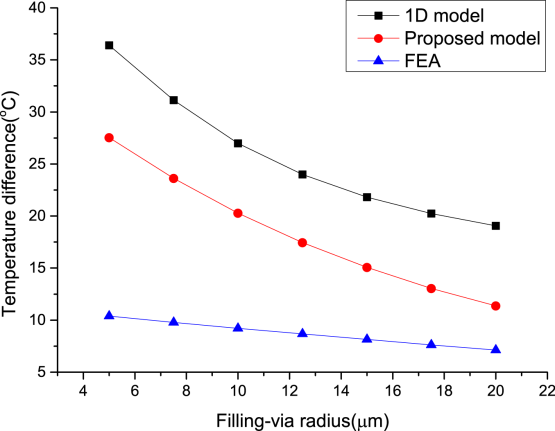Fig. 9. Temperature difference with respect to thickness of bulk Si. The radius of filling-via is 10 µm and the other parameters are d=1 µm, tb1=tb2=10 µm.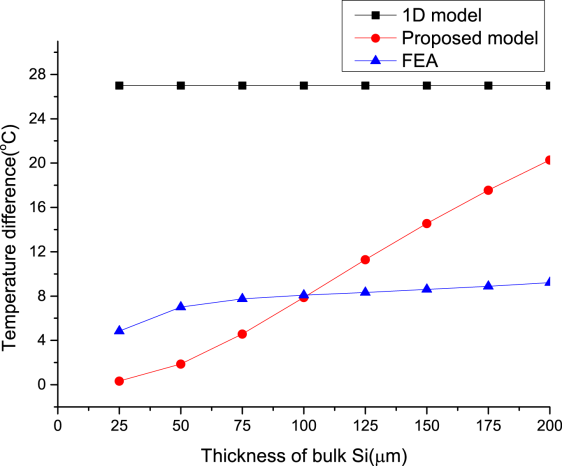In Fig. 9, both FEA result and proposed model show the temperature difference increases when the thickness of bulk Si increases. However,1D model shows only a little decrease where the increase of effective thermal conductivity can compensate the increase of the thermal length.

In Fig. 10, the proposed model finally approaches the FEA result while the 1D model almost remains constant because the effective thermal conductivity keeps nearly unchanged due to the tiny change of liner thickness.

As illustrated in Fig. 11, bonding layer thickness has a significant impact on the temperature rise and acts more like a thermal barrier between Si layers. The temperature rise increases as the bonding layer thickness goes up. Both the 1D model and proposed model show the same relationship while 1D model shows larger error when the bonding layer thickness goes down and the typical bonding layer thickness in 3D integration process is 10~${\mathrm{\mu}}$m.

Fig. 10. Temperature difference with respect to TSV liner thickness. The radius of filling-via is 20 µm and the other parameters are tb1=tb2=10 µm, H1=H2=200 µm.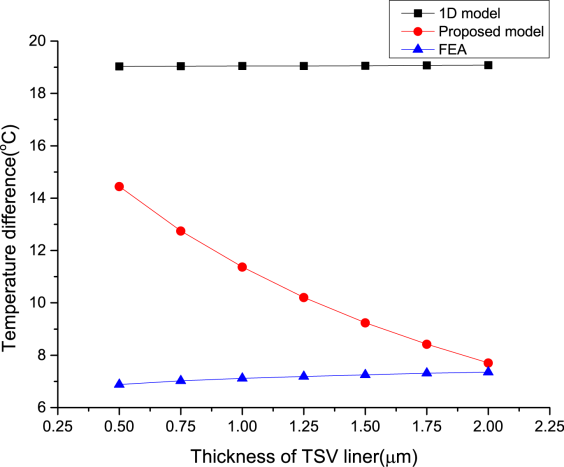Fig. 11. Temperature difference with respect to thickness of bonding layer. The radius of filling-via is 10 µm and the other parameters are d=1 µm, H1=H2=200 µm.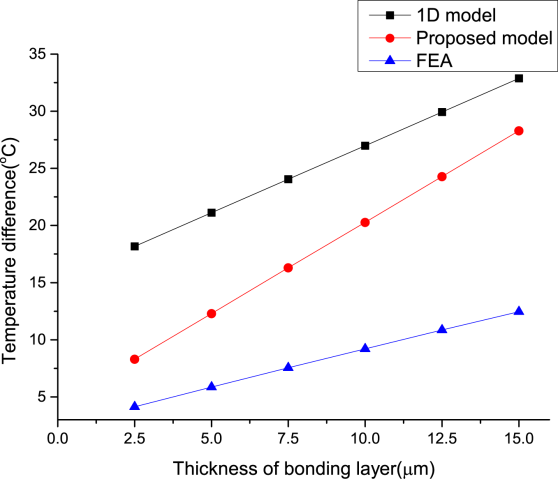Fig. 12 shows exact FEA model based on COMSOL software. The length and width are both set 500 µm, and each power cell consumes 1W of power which occupies 300 µm 300 µm area. Other parameters such as filling-via radius, thickness of bulk Si, TSV liner thickness and thickness of bonding layer are variables, which can be changed in every FEA simulation.

Fig. 13. 2-layers stacked power system using TTSVs as thermal mitigation tool.H1=H2=150 µm, tb1=tb2=10 µm, d=1 µm and the filling-via radius is 20 µm.## IV. CASE STUDY

The analytical model has been applied to a 3D stacked power system with internal TTSVs as a heat dissipation method. The construction of this 3D integrated system is shown in Fig. 13. The size for each power cell is 300 ${\mathrm{\mu}}$m ${\times}$ 300 ${\mathrm{\mu}}$m and generated power for each is 1W. The bottom surface of the heat sink made of copper is set constant temperature at 293.15K and the rest surfaces of the stacked structure are adiabatic which is close to the practical package case. The FEA simulation result shows that the steady state temperature of the top layer is 304.51K and for the bottom layer is 301.33K. The temperature rise is 3.18K between two layers due to the large filling-via radius and thinned bulk Si thickness. The analytical model gives 6.96K temperature rise and the 1D model has 10.17K temperature rise. Thus, the analytical model is superior than the 1D model in the proposed heat mitigation structure. Though FEA result is much more accurate but this method is extremely time consuming for chip scale numerical analysis. It takes less than 1 second for the analytical and 1D model, which is much shorter compared to the FEA method that consuming 7 minutes in a small computer workstation with 64GB memory.

## V. CONCLUSIONS

In summary, we have proposed a heat mitigation structure based on the consideration of the impact of TTSV thermal stress on the carrier mobility changes. We have shown the relationship between temperature difference in two layers and the filling-via radius, thickness of bulk Si, thickness of TSV liner as well as bonding layer thickness using the proposed analytical model and the 1D model without any curve fitting coefficients which can be extended to more than two layers situations. Both the FEA results and the proposed analytical model are helpful in the heat management design of 3D ICs and its reliability improvement.

### ACKNOWLEDGMENTS

This work was supported by National Natural Science Foundation of China under Grant 61664004 and 61464002, and supported by Science and Technology Project of Guizhou Province under Grant Qian Ke He Ping Tai Ren Cai 5788 hao, and supported by the Open Foundation of Engineering Research Center of Reliability of Semiconductor Power Devices of the Ministry of Education under Grant 010201.

### REFERENCES

1
Xu. X., Karmarkar. A., 2011, 3D TCAD Modeling For Stress Management In Through Silicon Via (TSV) Stacks, AIP Conference Proceedings, Vol. 1378, No. 1, pp. 53-662
Jiang. T., Wu. C. , Spinella. L. , Im. J. , Tamura. N. , Kunz. M. , Son. H.-Y., Kim. B. Gyu , Huang. R. , Ho. P. S. , 2013, Plasticity mechanism for copper extrusion in through-silicon vias for three-dimensional interconnects, Applied Physics Letters, Vol. 103, No. 21, pp. 2119063
Cheng H.-C., Huang T.-C., Hwang P.-W. , Chen W.-H. , 2016, Heat dissipation assessment of through silicon via (tsv)-based 3d ic packaging for cmos image sensing, Microelectronics Reliability, Vol. 59, pp. 84-944
Qiu D. , Cao L. , Wang Q., Hou F., Wang X. , 2017, Experimental and numerical study of 3d stacked dies under forced air cooling and water immersion cooling, Microelectronics Reliability, Vol. 74, pp. 34-435
Souare P. M. , Fiori V. , Farcy A. , de Crcy F. , Jamaa H. B. , Bor- bely A., Coudrain P. , Colonna J. P. , Gallois-Garreignot S. , Gi- raud B. , Cheramy S. , Tavernier C., Michailos J. , 2014, Thermal effects of silicon thickness in 3-d ics: Measurements and simulations, IEEE Transactions on Components, Packaging and Manufacturing Technology, Vol. 4, No. 8, pp. 1284-12926
Shin Y. , Kim S. E. , Kim S. , 2016, Thermal assessment of copper through silicon via in 3d ic, Microelectronic Engineering, mAM (Materials for Advanced Metallization), Vol. 156, pp. 2-57
Jain A. , Jones R. E. , Chatterjee R. , Pozder S. , Huang Z., 2008, Thermal modeling and design of 3d integrated circuits, in: 2008 11th Intersociety Conference on Thermal and Thermomechanical Phenomena in Electronic Systems, pp. 1139-11458
Ayala J. L. , Sridhar A. , Cuesta D. , 2010, Thermal modeling and analysis of 3d multi-processor chips, Integration, the VLSI Journa, Vol. 43, No. 4, pp. 327-3419
Wang F. , Yu N. , 2016, Thermal management of coaxial through- silicon-via (c-tsv)-based three-dimensional integrated circuit (3d ic), IEICE Electronics Express, Vol. 13, No. 11, pp. 20151117-2015111710
Liu Z., Swarup S. , Tan S. X. D. , 2013, Compact lateral thermal resistance modeling and characterization for tsv and tsv array, in: 2013 IEEE/ACM International Conference on Computer-Aided Design (ICCAD), pp. 275-28011
Liu Z. , Swarup S. , Tan S. X. D. , Chen H. B. , Wang H., 2014, Compact lateral thermal resistance model of tsvs for fast finite-difference based thermal analysis of 3-d stacked ics, IEEE Transactions on Computer-Aided Design of Integrated Circuits and Systems, Vol. 33, No. 10, pp. 1490-150212
Xu H. , Pavlidis V. F. , Micheli G. D. , 2011, Analytical heat transfer model for thermal through-silicon vias, in: 2011 Design, Automation Test in Europe, pp. 1-613
Huang B. , Wu Z. , 2017, The thermal analysis study of 3d bare chip laminated packaging structure, AIP Conference Proceedings, Vol. 1980, No. 1, pp. 04005414
Ryu S. K. , Lu K. H. , Jiang T. , Im J. H. , Huang R. , Ho P. S. , 2012, Effect of thermal stresses on carrier mobility and keep-out zone around through-silicon vias for 3-d integration, IEEE Transactions on Device and Materials Reliability, Vol. 12, No. 2, pp. 255-26215
Tsai M. Y. , Huang P. S. , Huang C. Y. , Jao H. , Huang B., Wu B. , Lin Y. Y. , Liao W., Huang J. , Huang L., Shih S., Lin J. P. , 2013, Investigation on cu tsv-induced koz in silicon chips: Simulations and experiments, IEEE Transactions on Electron Devices, Vol. 60, No. 7, pp. 2331-233716
Pi Y. , Wang W. , Jin Y., 2016, An accurate calculation method on thermal effectiveness of tsv and wire, in: 2016 IEEE 18th Electronics Packaging Technology Conference (EPTC), pp. 569-573## Author

Yongyong Wang received B.S. degree from Guizhou Normal University in Physics in 2016.

And he received M.S. degree from Guizhou University in Electronic Science and Technology in 2019.

In 2019, he joined Yangtze Memory Technologies Co.(YMTC), where he is involved in the design of CMOS periphery circuits for 3D stacked Nand Flash.

His main interests include analog/mixed signal circuit design and 3D integration technologies.

Kui Ma received the B.S. degree in electronics science and technology in 2007 and Ph.D. degree in micro-electronics and solid-state electronics in 2013, all from the Department of Electronics, Guizhou University, Guiyang, China.

He jointed Guizhou University as an Assistant Professor from 2013 to 2016, and has been an Associate Professor since 2017.

He worked as a visiting scholar at University of Toronto from 2016 to 2017.

His research interests include power semiconductor devices and integrated circuits, power integration technologies and reliability of power chips.

Dr. Ma was a recipient of the 2016 Chinese Government Scholarship for his study at the University of Toronto, Toronto, ON, Canada, from 2016 to 2017.

Kui Ma received the B.S. degree in electronics science and technology in 2007 and Ph.D. degree in micro-electronics and solid-state electronics in 2013, all from the Department of Electronics, Guizhou University, Guiyang, China.

He jointed Guizhou University as an Assistant Professor from 2013 to 2016, and has been an Associate Professor since 2017.

He worked as a visiting scholar at University of Toronto from 2016 to 2017.

His research interests include power semiconductor devices and integrated circuits, power integration technologies and reliability of power chips.

Dr. Ma was a recipient of the 2016 Chinese Government Scholarship for his study at the University of Toronto, Toronto, ON, Canada, from 2016 to 2017.